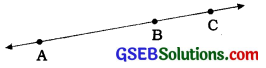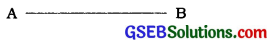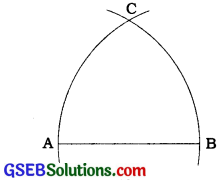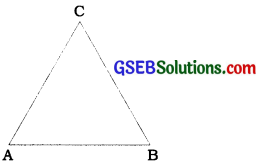# GSEB Class 9 Maths Notes Chapter 5 Triangles

This GSEB Class 9 Maths Notes Chapter 5 Triangles covers all the important topics and concepts as mentioned in the chapter.

## Triangles Class 9 GSEB Notes

Introduction to Geometry:
The word ‘geometry’ comes from the combination of two Greek words ‘geo’ meaning ‘the earth’ and ‘metrein’ meaning ‘to measure’. This branch of Mathematics was studied in various forms in every ancient civilization in Egypt, Babylonia, India, China, Greece, etc.

It is believed that the knowledge of geometry passed on from Egyptians to Greeks. A Greek mathematician Thales is considered to be the pioneer of geometry. Pythagoras was one of the most popular students of Thales.

Sulbasutras were created between 800 BC to 500 BC and that suggest that Indians knew the Pythagoras’ theorem before the birth of Pythagoras.

Euclid’s approach:
Geometry was being developed in unorganised manner in different civilizations. Euclid (325 BC to 265 BC) was a teacher of mathematics at Alexandria in Egypt. He collected all the known results and compiled them in a series of thirteen chapters each called a book. The compilation was named ‘Elements’. The notions of point, line, plane were derived from surrounding objects. An abstract geometrical concept of a solid object was created and developed from studies of space. Boundaries of a solid are surfaces. Surfaces have no thickness and their boundaries are curves or straight lines. The lines end in points. A point has no dimension.

 Geometric quantity Dimension Solid 3 Surface 2 Line 1 Point 0

Euclid gave 23 defInitions in Book 1 of the Elements.
A few of them are given below:

• A point is that which has no part.
• A line is breadthless length.
• The ends of a line are points.
• A straight line is a line which lies evenly with the points on itself.
• A surface is that which has length and breadth only.
• The edges of a surface are lines.
• A plane surface is a surface which lies evenly with the straight lines on itself.

But, these definitions led to a chain of terms which cannot or need not be defined. So, point, line, plane, etc. are taken as undefined terms.

Then, Euclid assumed certain properties which were not to be proved. He divided them into two types: axioms and postulates.

Axiom means a self-evident truth related to any branch of studies. Postulate means a stipulation or an assumption or a fundamental principle or a position assumed as self-evident related to studies of geometry.Some of Euclid’s axioms are given below:

• Things which are equal to the same thing are equal to one another.
• If equals are added to equals, the wholes are equal.
• If equals are subtracted from equals, the remainders are equal.
• Things which coincide with one another are equal to one another.
• The whole is greater than a part.
• Things which are double of the same thing are equal to one another.
• Things which are halves of the same thing are equal to one another.

Euclid’s five postulates are given below:

• A straight line may be drawn from any one point to any other point. Axiom 5.1: Given two distinct points, there Is a unique line that passes through them.
• A terminated line can be produced Indefinitely. Note: Euclid used the word termlnated line for ‘line segment’.
• A circle can be drawn with any centre and any radius.
• All right angles are equal tq one another.
• If a straight line falling on two straight lines makes the interior angles on the same side of It taken together Is less than two right angles then the two straight lines If produced Indefinitely meet on that side on which the sum of angles Is less than two right angles.

Using these axioms and postulates, Euclid proved many results which are known as propositions or theorems. Theorem 5.1: Two distinct lines cannot have more than one point in common.

Example 1:
If A, B and C are three points on a line, and B lies between A and C (see figure), then prove that AB + BC = AC.In the figure given above, AC coincides with AB + BC.
Also, Euclid’s axiom (4) says that things which coincide with one another are equal to one another. So, it can be deduced that AB + BC = AC
Note that in this solution, it has been assumed that there is a unique line passing through two points.

Example 2:
Prove that an equilateral triangle can be constructed on any given line segment.
In the statement above, a line segment of any length is given, say AB [see figure].Using Euclid’s postulate 3, we can draw a circle with point A as the centre and AB as the radius [see figure].Similarly, draw another circle with point B as the centre and BA as the radius. The two circles meet at a point, say C. Now, draw the line segments AC and BC to form A ABC [see figure below].Now, AB = AC, (Radii of the same circle) (1)
Similarly, AB = BC (Radii of the same circle) (2)
From these two facts, and Euclid’s axiom that things which are equal to the same thing are equal to one another, we can conclude that AB = BC = AC.
So, ∆ ABC is an equilateral triangle.

Theorem 5.1:
Two distinct lines cannot have more than one point in common.
Proof:
Here we are given two lines l and m. We need to prove that they have only one point in common.

For the time being, let us suppose that the two lines intersect in two distinct points, say P and Q. So, you have two lines passing through two distinct points P and Q. But this assumption clashes with the axiom that only one line can pass through two distinct points. So, the assumption that we started with, that two lines can pass through two distinct points is wrong.

From this, we are forced to conclude that two distinct lines cannot have more than one point in common.

Equivalent versions of Euclid’s fifth postulate:
Many mathematicians gave equivalent versions of this 5th postulate. One of them ‘Playfair’s axiom’ is as below: For every line l and every point P not lying on l, there exist unique line m passing through P and parallel to 1.
It means that two distinct intersecting lines cannot be parallel to the same line.
Among the Indian mathematicians Arya- bhatta, Brahmgupta and Bhaskaracharya are the chief architects of geometry.

Non-Euclidean geometry: Many mathema-ticians, including Euclid, belived that 5th postulate is a theorem which can be proved using logical reasoning. But all attempts to prove the 5th postulate have failed. But, these efforts led to creation of several other geometries known as ‘non Euclidean geometries’. One Important non. Euclidean geometry Is ‘Spherical geometry’. In spherical geometry; lines are not straight. They are parts of great circles. In spherical geometry, the sum of angles of a triangle Is greater than 1800. Euclldean geometry Is valid only for the figures In the plane. On the curved surface, It fails.Example 1:
Consider the following statement:
There exists a pair of straight lines that are everywhere equidistant from one another. Is this statement a direct consequence of Euclid’s fifth postulate? Explain.